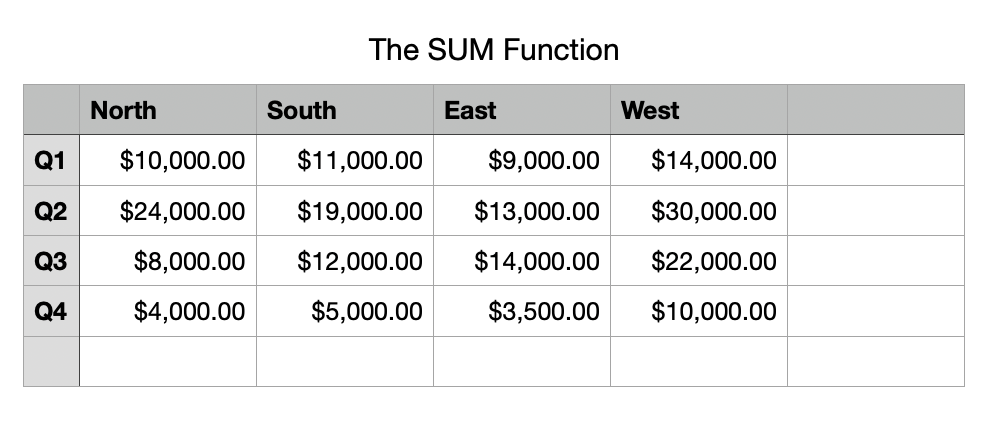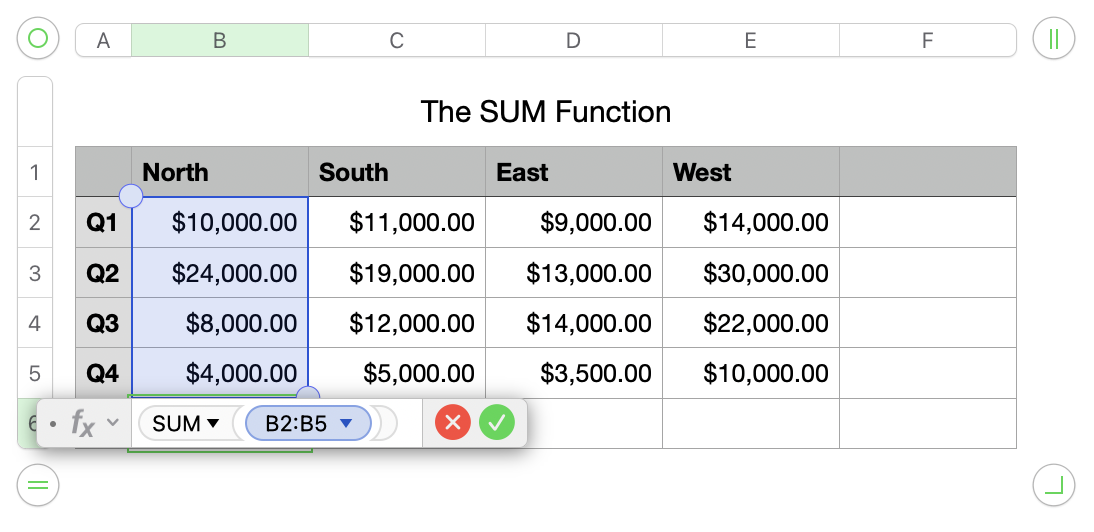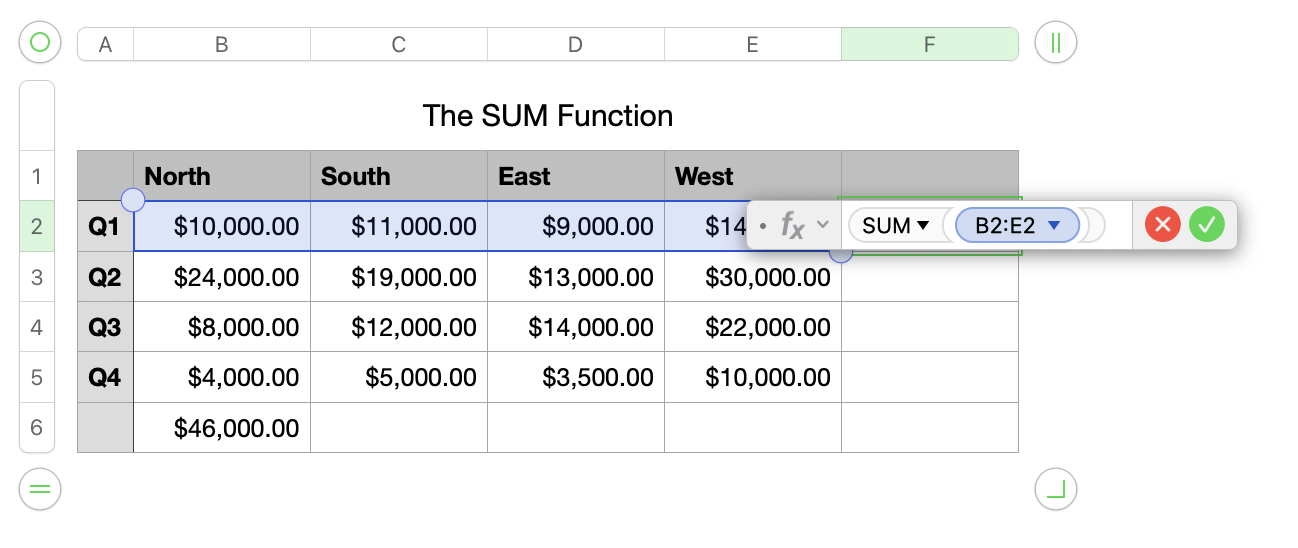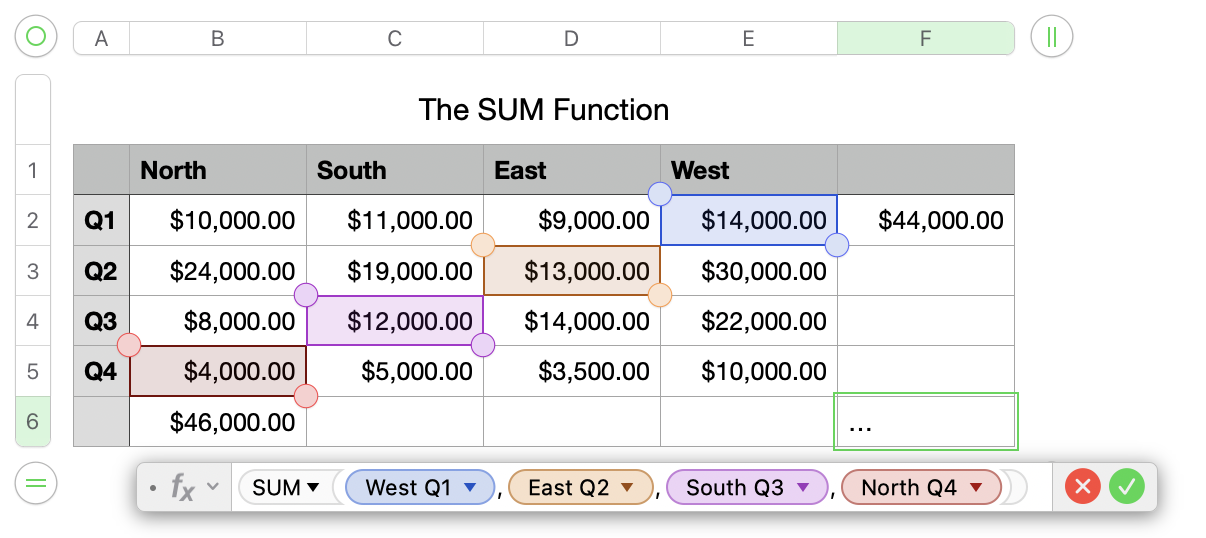Numbers is a powerful spreadsheet software. It has gone through a lot of change since I first started using it back in 2008. But the SUM function has been through it all.

If you are looking for a spreadsheet software, Numbers is an excellent option. Its great for users of all skill levels. And the SUM function or its derivatives is probably the most widely used function in Numbers so it’s a great idea to have a good handle on how to use it. In this article we are going to take a deep dive into how to use the Numbers SUM function and why you would want to use it.

Spreadsheets have a long history of allowing users to quickly make calculations to help them understand their raw data. The SUM function is about one of the most useful functions in the world of spreadsheets. Simply put the SUM function allow you to get the sum to 2 or more cells.

The SUM function would look something like this depending on your application:

=SUM(A1:A5)

Let’s dive into the uses for the SUM function.

## How to Use the SUM Function

Before we dive into how to use the SUM function we should first mention that if you aren’t familiar with using functions please check out my other article – Using Functions in Numbers – before you start. It will add some much needed context.

In the early days of spreadsheets users were constantly trying to do simple math calculations across different cells and that would have looked something like this:

=A1+B1+C1

And while that worked and you can still do it today developers have created the SUM function to make it simpler, and remove much of the syntax or uneccesary charaters to make it quicker to do calculations.

Now that same function could look like this:

=SUM(A1:C1)

Although the first has fewer characters you can start to see the ease of use the SUM function can add to spreadsheets.

Let’s take a look at how you can use the SUM function in your spreadsheets.

## How to SUM a Column In Numbers

The SUM function has a lot of uses but one trick up its sleeve is that it will allow a user to get the SUM of a set of numbers in a column. It can do it a couple of ways. Let’s take a look at them.Let’s use this small sample spreadsheet as an example. This spreadsheet has some example sales figures for a fictional organization. There are 2 main ways that you could use the SUM function to get the sales totals of a column.

First, highlight cell B6 underneath the \$4,000 figure. We are going to get the total of sales for the North Region. Second, type the following, “=SUM(B2,B3,B4,B5)“. This will add the values of those cells.

The second way you could do it is by clicking and dragging the cells you wanted to include in the function. First type, “=SUM(“, then click on cell B2 and drag through cell B5, then type, “)”, and hit the “return” key. This will be displayed as “=SUM(B2:B5)” as shown below.Now, let’s move on and talk about more uses of the SUM function.

## How to SUM a Row in Numbers

Similar to getting the sum of a column calculating the sum of a row the SUM function works in the same mannor. Let’s take a look at our example sheet.If I place my cursor in cell F2 and press the equal “=” key, it will bring up this special functions window and help me to write a function. Then I’ll type, “SUM(B2:E2)”, and press the “return” key and Numbers will give me the sales totals for Q1. You can also click into cell B2 and drag your cursor the cell E2 to select your cells to sum.

Like with a column, you can also enter a comma seperated list of the cells you wish to sum together that would look like this:

=SUM(B2,C2,D2,E2)

This is an extremely common way to calculate large amounts of data in a single row quickly.

## Other Ways to Use the SUM Function

There are a couple of other ways that you might use the SUM function that you should be aware of.

### Calculate a Comma Seperated List of Cells

First, I’ve alluded to to a couple of times. With a comma seperated list of cells in the SUM function you can get the total of any cells with numbers that you want.While this example doesn’t make a lot of sense and I wouldn’t need to do this for this scenario, you can see the benefits. All I did was type the equal sign “=SUM(” and then clicked onto the cells that I wanted to include in the function. Pressing the “return” key will give me the calculated results.

### Nesting the SUM Function

The SUM function can be nested inside another function to make for some more complex calculations. Say for instance you wanted to get the average sales per quarter for the entire company. Well, one way you could it would look be this:

=AVERAGE(SUM(B2:B5),SUM(C2:C5),SUM(D2:D5),SUM(E2:E5))

In conclusion, the SUM function in Numbers is a powerful tool that simplifies calculations and enhances the efficiency of spreadsheets. Whether you need to calculate the sum of a column or a row, or even perform more complex calculations by nesting the SUM function, Numbers provides a user-friendly interface to accomplish these tasks. By selecting the cells you want to include in the function, you can easily obtain accurate results with just a few simple steps. Embrace the convenience and ease of use that the SUM function offers, and take your spreadsheet calculations to the next level.